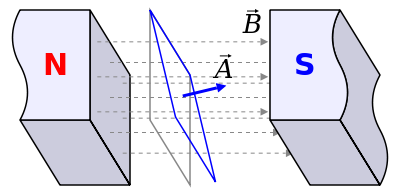# Magnetic flux

Magnetic flux is the amount of magnetic field perpendicular to a surface, passing through that surface. Changing magnetic flux creates an electromotive force, and if near a wire makes electric current flow through an electric circuit.

A full treatment of this subject is necessary to understand the internal workings of electric generators and electric motors, but the physics and mathematics behind magnetic flux are beyond the scope of this encyclopedia and take years of study to understand properly. An excellent place to start is hyperphysics, but D. J. Griffiths' Introduction to Electricity and Magnetism is an excellent text on the subject.Figure 1. The magnetic flux is the field going through the area. Note that if the field isn't perpendicular to the plane of the loop of wire, one has to take the component perpendicular by multiplying by the cosine of that angle.